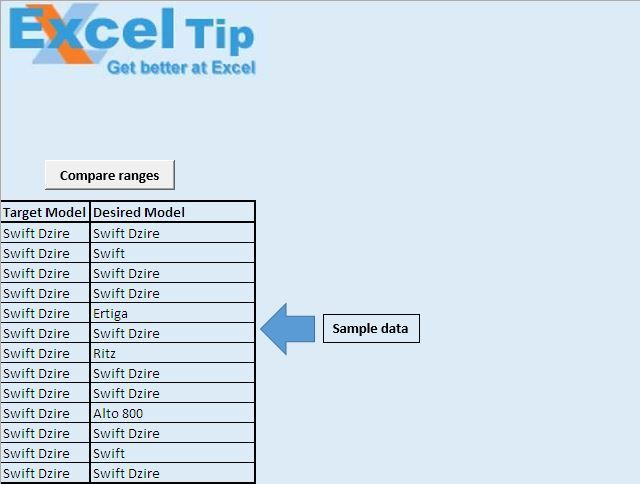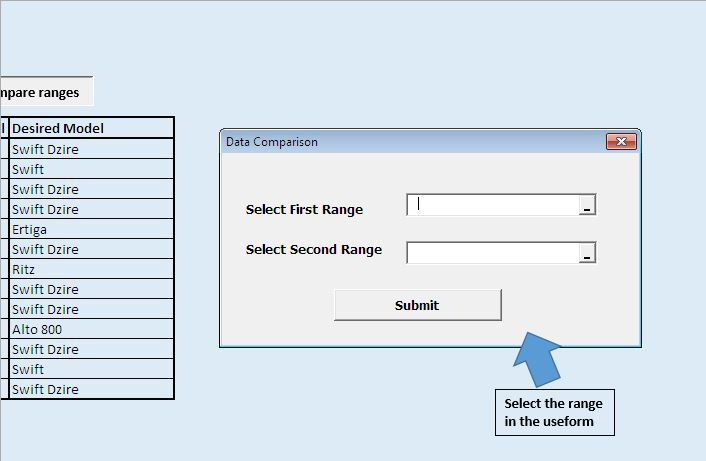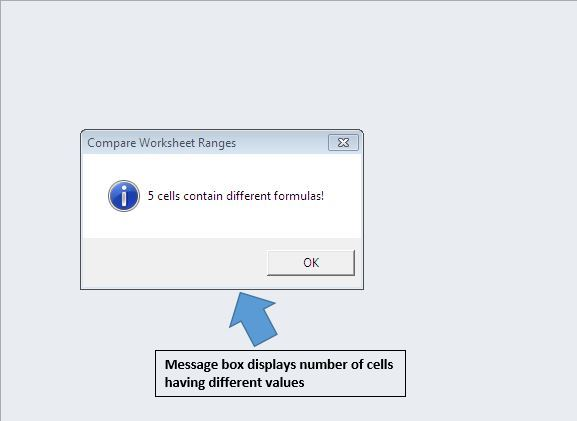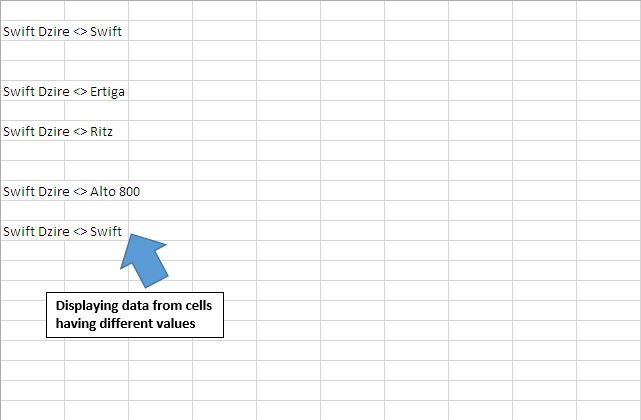# Compare two worksheet ranges using VBA in Microsoft Excel

In this article, we will create a userform to compare two ranges and find out non-matching cells.

Raw data consists the data of target models and desired models. We want to find those records in which target and desired models are not matching.We have created a userform which accepts two ranges as input. These two ranges are compared to find non-matching cells.On clicking the submit button, it will compare two ranges and return output. It will display a message box, displaying the count of non-matching cells.It will also give data on non-matching cells in a new workbook.Code explanation

Set Rng1 = Range(UserForm3.RefEdit1)

The above code is used to create an object of range object, which gets range values from the userform.

If Rng1 Is Nothing Or Rng2 Is Nothing Then Exit Sub

The above code is used to check whether both ranges contain values. If any of the ranges is left blank, then it skips rest of the code within the procedure.

With Rng1

LR1 = .Rows.Count

LC1 = .Columns.Count

End With

The above code is used to get the count of number of rows and columns within the range.

CellValue1 = Rng1.Cells(r, c).FormulaLocal

The above code is used to get the value in the cell of r row and c column.

If CellValue1 <> CellValue2 Then

The above code is used to compare values in variables CellValue1 and CellValue2.

```
Option Explicit

Sub CallingUserform()

UserForm3.Show

End Sub

'Insert below code in userform
Option Explicit

Private Sub CommandButton1_Click()

'Declaring variables
Dim Rng1, Rng2 As Range
Dim r, DiffCount As Long, c As Integer
Dim LR1 As Long, LC1 As Integer
Dim CellValue1 As String, CellValue2 As String
Dim NewWB As Workbook

'Getting the two range set for comparing
Set Rng1 = Range(UserForm3.RefEdit1)
Set Rng2 = Range(UserForm3.RefEdit2)

Application.ScreenUpdating = False

'Checking whether Rng1 and Rng2 contains value
If Rng1 Is Nothing Or Rng2 Is Nothing Then Exit Sub

'Getting count of number of rows and columns in Rng1
With Rng1
LR1 = .Rows.Count
LC1 = .Columns.Count
End With

DiffCount = 0

'Adding new workbook for output
Set NewWB = Workbooks.Add

'Looping through all the columns and rows in the range
For c = 1 To LC1
For r = 1 To LR1

'Getting value from particular cell from both the ranges
CellValue1 = Rng1.Cells(r, c).FormulaLocal
CellValue2 = Rng2.Cells(r, c).FormulaLocal

'Comparing value of cell from both ranges
If CellValue1 <> CellValue2 Then

'Getting count of numbers of cells with different values
DiffCount = DiffCount + 1

'Adding unequal values to new workbook
Cells(r, c).Value = "'" & CellValue1 & " <> " & CellValue2
End If

Next r
Next c

'Display count of unequal cells in both range
MsgBox DiffCount & " cells contain different formulas!", _
vbInformation, "Compare Worksheet Ranges"

Application.ScreenUpdating = True

Set NewWB = Nothing

End Sub

```

If you liked this blog, share it with your friends on Facebook. Also, you can follow us on Twitter and Facebook.

We would love to hear from you, do let us know how we can improve our work and make it better for you. Write to us at info@exceltip.com

1.Set R1 = Range(S1.Cells(1, 1), S1.Cells.SpecialCells(xlCellTypeLastCell))
Set R2 = Range(S2.Cells(1, 1), S2.Cells.SpecialCells(xlCellTypeLastCell))
S3.Cells(1, 1).Formula = "=" & R1.Address(, , , True) & "=" & R2.Address(, , , True)
bComp = S3.Cells(1, 1)

•To be more clear....for all the cells comparing...

Set R1 = Range(S1.Cells(1, 1), S1.Cells.SpecialCells(xlCellTypeLastCell))
Set R2 = Range(S2.Cells(1, 1), S2.Cells.SpecialCells(xlCellTypeLastCell))
If R1.Count = R2.Count Then
Set R3 = Range(S3.Cells(1, 1), S3.Cells(S2.Cells.SpecialCells(xlCellTypeLastCell).Row, S2.Cells.SpecialCells(xlCellTypeLastCell).Column))
R3.Formula = "=" & R1.Address(, , , True) & "=" & R2.Address(, , , True)
Set R = R3.Find(What:="FALSE", After:=S3.Cells(1, 1), LookIn:=xlValues, _
LookAt:=xlWhole, SearchOrder:=xlByRows, SearchDirection:=xlNext, _
MatchCase:=True, SearchFormat:=False)
bComp = R Is Nothing
Else
bComp = False
End If

2.what exactly do I but in VBn and what do I put in the actual worksheet?

Terms and Conditions of use

The applications/code on this site are distributed as is and without warranties or liability. In no event shall the owner of the copyrights, or the authors of the applications/code be liable for any loss of profit, any problems or any damage resulting from the use or evaluation of the applications/code.